Non-Euclidean dissimilarities may arise naturally when we want to build a measure that incorporates important knowledge about the objects. This is fine, but how to embed such dissimilarities in a vector space if we want to use the standard linear algebra tools for generalization? Here the so-called pseudo-Euclidean vector space will be discussed.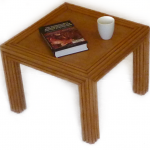To understand the problem, have a look at the picture to the right. There are three objects, a table (T), a book (B) and a mug (M). The book touches the table and, thereby the distance between the book and the table is 0. The same holds for the mug and the table. The book and the mug, however, lay apart. Let us say that they have a distance of 10. The entire pairwise distance matrix is thereby: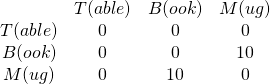It is clearly non-Euclidean. It is even non-metric as the triangle inequality does not hold. For instance, the book and the table have a distance 0, but they have different distances to the mug. So, if we find a vector space in which objects are represented as vectors which perfectly reflect the original distances, the distance measure used in that space cannot be Euclidean.

The following property will be very helpful to construct such a space. Let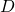be a symmetric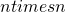dissimilarity matrix with non-negative values and zeros on the diagonal, then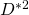can be split in two matrices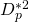and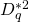by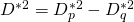such that both,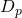and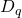are perfectly embeddable in an Euclidean space. In this notationmeans that all elements inare squared, i.e.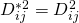. If the dimensionalities of these spaces are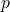andthen. The sizes ofandare of course. Note that any Euclidean dissimilarity matrix betweenvectors can be embedded in a ()-dimensional space.

The above equation can be interpreted as follows. There exists an Euclidean vector space of dimension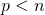in whichpoints can be positioned such that their Euclidean distances are equal or larger than the given pairwise dissimilarities in. If all corrections are computed according tothen the resulting set of dissimilaritiesappears to be Euclidean. For the above example the, so-called, p-space can be a one-dimensional space in which the book, the table and the mug are positioned on a straight line with the distances of 5:

The distance between the book and the mug is correct. Their distances to the table, however, needs some correction. Substitution of these distances in the above formula yields (after squaring the values):This shows that for the correction the, so-called, q-space, is necessary in which the distance between the book and the mug is 0 and both have a distance of 5 to the table. This is possible by the below 1-dimensional space.

The two spaces can be combined into a single 2-dimensional vector space. This space is still a vector space, but non-Euclidean as the distances should now be computed in the way discussed above. It is a Caresian product of two Euclidean spaces with a specific inner product and the distance. It is called a pseudo-Euclidean space and the two related subspaces are called the positive space (the p-space) and the negative space (the q-space). Below this space is shown for the discussed example.

The representation of the table is positioned in the origin of this space. The book and the mug are positioned on the diagonals and both have the same distance (5) to the table in the positive (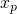) as well as in the negative () direction. Their total distance to the table is thereby 0.

What is interesting is that the shaded area cannot contain any vector (point) as its squared distance to the table (T) would be negative.

In this example two 1-dimensional spaces are combined together to yield the final space. In practical situations both sub-spaces will be multidimensional and the resulting vector space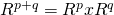is-dimensional. However, the definition of classifiers not straightforward because of the specific distance measure. Classifiers based on the point-wise distances such as the nearest neighbor rule and the Parzen classifier can be used here, but probability density functions are in general not well defined. Also classifiers based on distance optimization such as the support vector machine cannot be used here without a through consideration.

A significant problem of the application of pseudo-Euclidean spaces is that the projection of new objects to the existing space is not well defined. It is certainly possible based on the algebra of the inner products, but it may come into a conflict when one wants to minimize distances (or projection error). In a pseudo-Euclidean space the (squared) distance can be negative. The original distance of a projection is thereby not applicable.

Filed under: EmbeddingPR System